# Simplify Surds Using Square Factors

In this worksheet, students will simplify surds into multiples of square roots and square factors.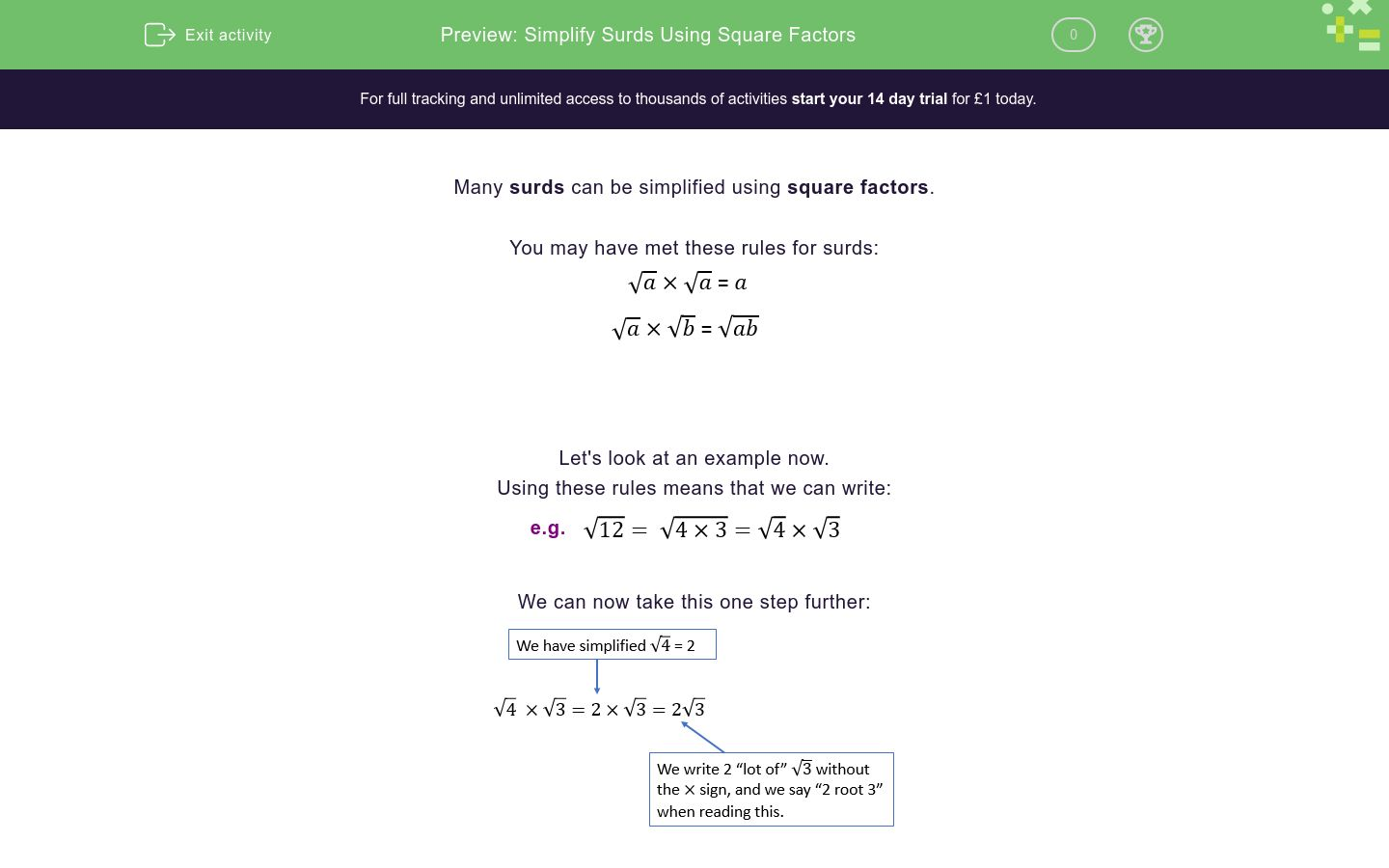Key stage:  KS 4

GCSE Subjects:   Maths

GCSE Boards:   AQA, Eduqas, Pearson Edexcel, OCR

Curriculum topic:   Number, Indices and Surds

Curriculum subtopic:   Structure and Calculation, Exact Calculations

Difficulty level:### QUESTION 1 of 10

Many surds can be simplified using square factors.

You may have met these rules for surds:Let's look at an example now.

Using these rules means that we can write:

e.g.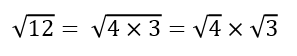We can now take this one step further: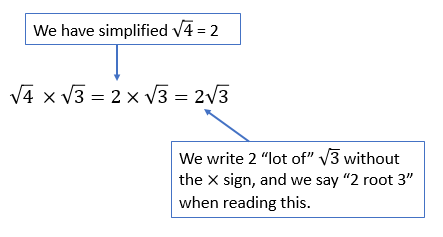We will need to look for square factors of any surd we are asked to simplify.

Here is another example.

e.g. Simplify:We need to think about the factors of 20.

They are 1 x 20, 2 x 10 and 4 x 5.

We know that 4 is a square number and so it would be helpful to rewrite √20 as 4 x 5.

So we can simplify as follows:In this activity, we will simplify surds into multiples of square roots.

This will be a useful skill to support your mental maths and problem solving in all areas of maths.

You may want to have a pen and paper handy so that you can record your working and compare these to our examples written by a teacher.

Simplify:

√28

Hint:
Remember to think about the factor pairs of 28 and choose a pair which involves a square number.

∜7

2√7

7√2

Cannot be simplified

Simplify:

9√5

5√2

∛5

Cannot be simplified

To make sure that we simplify a surd as completely as possible in as few steps as possible, we need to make sure we select the largest square factor.

e.g. Simplify:

Now we can write this as:However, although this is a simplification of the surd, it's not a complete simplification because &radic;12 can itself be simplified.

So the correct way to fully simplify &radic;48 is:48 has factors that include the square numbers 4 and 16, but we should work with the largest option in general.

Here's one for you to try now.

Fully simplify:

2√20

4√5

5√4

Cannot be simplified

You can be asked to simplify a surd in another way too.

Type the value of k as a number below.

We can also be asked to simplify multiples of square roots.

e.g. Simplify 2√9.Therefore, the 'simplest form' of a square root may be a single integer like this.

Now give this a try yourself.

Simplify:

2√25

50

25

10

52

We can also be asked to put the last two steps together, and simplify surds using both multiples and whole numbers.

e.g. Simplify 2√45.Now give this a try yourself.

Express 2√75 in the form k√3 where k is an integer.

Type the value of k as a number below.

It can be useful to be able to reverse this process too.

We can take a simplified surd and write it as a integer under the square root sign.

e.g.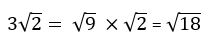It's your turn to give this reverse process a try now.

Which of the options below is equivalent to <sup>4</sup>&radic;3?​

√48

√12

√36

√144

Type the value of k as a number below.

For an extra challenge,let's try working with algebraic variables in surds.

Simplify:

Which of the options below accurately represents the answer?

ab

a√b

Cannot be simplified

Consider this term:

c2√d

When you write this term as a single root, what power, index or order is attached to the letter c?

• Question 1

Simplify:

√28

Hint:
Remember to think about the factor pairs of 28 and choose a pair which involves a square number.

2√7
EDDIE SAYS
What multiples can 28 be split into? 1 x 28, 2 x 14, 4 x 7 Which of these options contain a square number? Only 1 and 4 are square numbers in these options. Which would create a simpler option? If we follow this working through, it should look something like this: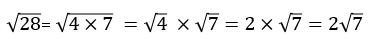• Question 2

Simplify:

∛5
EDDIE SAYS
Which multiples can be split 45 into? 1 x 45, 3 x 15, 5 x 9 Which of these options contain a square number? Only 1 and 9 are square numbers, but 1 cannot be used to create a simpler expression as it will stay the same. Therefore, let's work with the sum: 5 x 9 If we follow the working through, it should look something like this:• Question 3

To make sure that we simplify a surd as completely as possible in as few steps as possible, we need to make sure we select the largest square factor.

e.g. Simplify:

Now we can write this as:However, although this is a simplification of the surd, it's not a complete simplification because &radic;12 can itself be simplified.

So the correct way to fully simplify &radic;48 is:48 has factors that include the square numbers 4 and 16, but we should work with the largest option in general.

Here's one for you to try now.

Fully simplify:

4√5
EDDIE SAYS
Although the first option is a simplification of the surd, we need to use the largest square factor to simplify fully. Let's list the possible factors of 80: 1 x 80, 2 x 40, 4 x 20, 5 x 16, 8 x 10 Our options involving square numbers are: 1 x 80, 4 x 20, 5 x 16 Therefore, 16 is the highest square number we can choose to work with. If we follow this process through using 5 and 16 as our factors, our working will be:• Question 4

You can be asked to simplify a surd in another way too.

Type the value of k as a number below.

5
EDDIE SAYS
We need to follow the same process here. Our multiples are: 1 x 75, 3 x 25, 5 x 15 Of these, only 1 and 25 are square numbers. So √75 = √25 x 3 = 5√3 In the format k√3, the number 5 represents 'k'.
• Question 5

We can also be asked to simplify multiples of square roots.

e.g. Simplify 2√9.Therefore, the 'simplest form' of a square root may be a single integer like this.

Now give this a try yourself.

Simplify:

2√25

10
EDDIE SAYS
Start by splitting the question into its whole number and root components. What is √25? That's right, it's the same as 5 because 5 x 5 = 25. Usually these questions will involve finding the root of square numbers which we commonly see - can you remember the first 10 square numbers? Our working should look like this: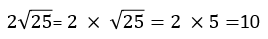• Question 6

We can also be asked to put the last two steps together, and simplify surds using both multiples and whole numbers.

e.g. Simplify 2√45.Now give this a try yourself.

Express 2√75 in the form k√3 where k is an integer.

Type the value of k as a number below.

10
EDDIE SAYS
Firstly, split the root into its two elements as we did in the previous question. Then work out how the number in the root (75) can be written as a product of two factors, where at least one of them is a square number. Here is an example of correct working for this question: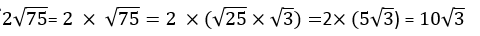• Question 7

It can be useful to be able to reverse this process too.

We can take a simplified surd and write it as a integer under the square root sign.

e.g.It's your turn to give this reverse process a try now.

Which of the options below is equivalent to <sup>4</sup>&radic;3?​

√48
EDDIE SAYS
So, simply speaking, we need to bring the whole number outside the root, back inside the root. We achieve this by squaring the number. 4 x 4 = 16 If we follow this working through, we see that:• Question 8

Type the value of k as a number below.

63
EDDIE SAYS
To move the 3 back under the root, we need to square it. 3 x 3 = 9 Here is the correct working for this question:• Question 9

For an extra challenge,let's try working with algebraic variables in surds.

Simplify:

Which of the options below accurately represents the answer?

a√b
EDDIE SAYS
First, we need to split &radic(a2b) into its two components. Did you spot that a 2 and a √ cancel each other out? Here is how we can work out the correct answer:• Question 10

Consider this term:

c2√d

When you write this term as a single root, what power, index or order is attached to the letter c?

4
EDDIE SAYS
c is already squared outside the root, so, to bring it inside, we need to square it again. Amazing work completing this activity! Why not try another activity on surds if you would like more practise? Here is the correct working for this question:---- OR ----

Sign up for a £1 trial so you can track and measure your child's progress on this activity.

### What is EdPlace?

We're your National Curriculum aligned online education content provider helping each child succeed in English, maths and science from year 1 to GCSE. With an EdPlace account you’ll be able to track and measure progress, helping each child achieve their best. We build confidence and attainment by personalising each child’s learning at a level that suits them.

Get started